Abstract. Dirk Huylebrouck and Patrick Labarque try to provide a positive answer to the question that the golden section corresponds to an optimal solution. It is but a college-level rephrasing exercise, but it could reboot the mathematical career of the golden section. An extension to the related silver section is given as well. The authors betin their examination with the definition of the golden number, then proceed to its applications to architecture, grey-tone mixing, colour mixing and bicycle gears.

#More True Applications of the Golden Number

Dirk Huylebrouck and Patrick Labarque
Sint-Lucas Institute for Architecture.
Paleizenstraat 65, 1030 Brussels BELGIUM

INTRODUTION
The metallic means are the positive roots of x2 - nx -1 = 0, for different values of the positive integer n. The first (n =1) is the most prominent member, the golden mean f, while for n = 2 the quadratic yields sAg the silver mean and the sBr bronze mean for n = 3 . Literature describes their properties extensively, often including wild statements of doubtful verification, repeated over and over, based on identical erroneous sources. Some false applications are simply results of a pseudoscientific new age imagination, while others build on approximate measurements allowing any desired interpretation. The so-called omnipresence of the mythical divine proportion should be assessed critically.

Nevertheless, colour theory already forced the authors to recognise a new true application of the metallic means. A partitive mixing of 2 complementary colours is "well-balanced and pure" if rotation of a disk covered for 50% each by these colours yields a 50% grey. Yet, the colour or grey promised by the software is rarely the one of the final output. It turns out that proportions of grey tones related to the metallic means play interesting roles in partitive mixing. This will be described in Section 2, and Section 3 will show extensions of these results to "golden colours", whose Red, Green, and Blue components are each of the proportion 1/f. Some related computational recreations illustrate this colourful theory.

More recently, the authors were again surprised to come across the golden number, in the completely different field of the determination of gears and the addition of speeds. This will be described in Section 4. Together with the colour theory applications, it shows that the appearance of the golden number is not always the result of a far-fetched imagination, like many statistical and historical 'studies' about the golden number. For instance, it has often been repeated, with dubious justification, that the rectangle of width 1 and length is considered as the most elegant one. Yet, in Section 5 we will answer a question concerning the optimality of the golden rectangle in artistic paintings or buildings' facades. This could reboot the mathematical career of the metallic means.

1. THE GOLDEN NUMBER
T
he golden number (or golden section, mean, or ratio, or divine proportion, etc.) arises when a line segment of length x (>1) is divided into two pieces of lengths 1 and x-1, such that the whole length is to 1, as 1 is to the remaining piece, x-1. Thus, the x / 1 ratio must equal the ratio 1/(x-1). This produces the equation x2 - x -1 = 0, of which f = (1+Ö5)/2 »1.6180 is the positive solution; note that f-1= 1/f »0.6180. More generally, the positive roots of x2 - nx -1 = 0 yield the family of metallic means, for n=1, 2, .... For n=2, the positive root is the silver mean sAg = 1+Ö2; for n=3, it is the bronze mean sBr = (3+Ö13)/2, etc.

Literature often describes the golden section as a cookbook-like formula. The historical justification is but a recipe: the (unequal) division of a line such that the whole length stands to the larger piece, as the larger is to the smaller. The authority of Euclid may be a good reason for using this recipe, which was termed simply "division in extreme and mean ratio". What importance Greek mathematicians attached to this number is unknown, and after all, the adjective "golden" dates from only 1844 [Markowsky 1992: 4]. The properties of these numbers have been described extensively (for a comprehensive survey, see [Spinadel 1998]). Of course, half the diagonal of a rectangle with length 2 and width 1 corresponds to the 'irrational part', Ö5/2, of the golden section, but this is so obvious that it is hardly a "mathematical application". The only related, straightforward yet surprising geometric properties are perhaps those of the pentagon. Therefore, the related angle of 36° is perhaps appropriately called the "golden angle" (Figure 1).

Some authors contend that the golden number is "the most irrational of all irrational numbers", with the other metallic means following in order of decreasing irrationality, because their representations in continued fraction form are the slowest converging. However, this justification does not use the conventional mathematical approach, as it does not specify when a number is more or less irrational than another number. To understand this, note that since x2 - x -1 = 0 can be rewritten x=1+1/(1+1/x), replacement of x by its expression in the denominator yields x=1+1/(1+x), which, when repeated ad infinitum, yields the continued fraction form for the solution,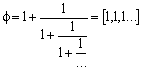,

and similarly,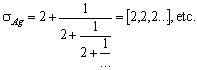Continued fractions converge more quickly when the successive denominators are large, but since the continued fraction expansion for f always involves adding only a 1 (the smallest possible positive integer) to the denominator, it converges the slowest (cf. [Spinadel 1998: 3-8]). While this may be important in some contexts, it is by no means standard to judge the "irrationality" of a number by the rate of convergence of its continued fraction expansion.

The lack of straightforward mathematical reasons to justify a prominent status of the golden section forces some to evoke aesthetical, historical, or natural considerations. The golden rectangle of width 1 and length f would be the most elegant one, as various designs would show. Yet, strong arguments show that the Greeks did not use it deliberately in their Parthenon (Figure 2), nor was the Great Pyramid necessarily designed to conform to f. Certainly Herodotus did not mention such a description; it simply does not appear in his text, as anyone can check. Nor does Leonardo's early work (nor the UN building, nor the human body, nor Virgil's Aeneid) constitute evidence of the golden ratio's exceptionality [Markowsky 1992]. Finally, the often-cited association of the golden rectangle spiral to that of the nautilus shell is seen to be incorrect upon a more thorough analysis [Sharp 2002].

Still, the Fibonacci sequence

1, 1, 2=1+1, 3=2+1, 5=3+2, 8=5+3, 13=8+5, …

is linked to f, since the quotient sequence, formed by taking the quotient of each term divided by the previous one, tends to f. Painters and sculptors often subdivide their (rectangular) canvas or stone using specific proportions, and 3 : 5 or 5 : 8 arrangements are frequent. This provides a connection to the Fibonacci numbers. Yet, 2 : 4 : 8 schemes are popular too and even used by great masters such as Seurat, Monet and Cézanne. It would be hard to prove that Fibonacci subdivisions lead to the "most beautiful" artworks. Furthermore, some contradiction is involved in the statement that golden section subdivisions are the key to fine art creations - why then would artists and their creative skills still be needed instead of a golden section computer program?

In the last resort, if no mathematical, aesthetical or historical reasons help, some turn to statistics. Yet, Markowsky asserts that the statistical experiments about the golden rectangle do not seem to confirm a preference for the golden rectangle. It is not indisputable that one can pick out the "most elegant" (golden) rectangle from an arbitrary list. Other examples seem mere repetitions of the same unreliable statistical studies. Matila Ghyka was one of the prominent advocates of the golden section myth, and his popular books influenced many readers, such as Le Corbusier. Of course, there is nothing wrong with the fact that architects use mathematics or arithmetical proportions as inspiration, instead of some romantic, ecological, ergonomic or other kind of muse to create their works of art. It even puts mathematics in a position of great honour that someone like Le Corbusier uses it. Nevertheless, these claims are artistic ones, without scientific consequences.

In spite of the vigorous considerations in disfavour of the golden section, many mathematicians continue to spread the myth. Mathematicians may be somewhat biased about the subject, maybe because they appreciate the attentions artists want to pay them, for once. A moderate conclusion comes from a psychologist, Christopher D. Green:

Although many researchers have concluded that the effect is illusory, more carefully conducted studies have fairly consistently shown that there is, in fact, a set of phenomena that require explanation, though no one has yet produced an explanation, both adequate and plausible, that has been able to stand the test of time. [Green 1995]

Nevertheless, we believe two cases can be added to the (not so lengthy) list of true applications of the golden section. The first is about colour theory, while the second stems from still another field, i.e., the study of planetary gears. In addition, the last section below concerns a classical mathematical justification for the interpretation of the golden section as an optimal solution, despite the previous critical considerations. Thus, maybe there is something more to be discovered about that (in)famous golden section.

2. MIXING GREY TONES
I
n much computer-graphics software, the user can numerically determine the kind of grey to use in shading a surface. Actually, the W-value of a grey surface indicates the amount of white in it (in most software, the amount of black is used instead). Thus, a W-value of 0 corresponds to black, while a W-value of 1 means in fact white, or, more precisely, "maximum light". Unfortunately, the value given by the software does not necessarily guarantee an identical result by the hardware. In the graphical sector, the determination of the exact grey value of a drawing is important, and some designers apply several transparent layers on top of each other on a white background to study this problem. Obtaining white through two layers corresponds to having white on the first AND on the second. Thus, appealing to probability theory, if W1 is the W-value of the first layer, and W2 the value of the second, their product provides the resulting W-value of the double layer. (Given two independent events A and B, the probability of both A and B occurring is given by their product: Pr(A and B)=Pr(A)Pr(B).) The illustration shows such a subtractive mixture (see the upper portion of Figure 3).

Rapidly rotating a disk with two different grey tones is another technique to judge the final impression of a black and white mixture. At each moment, a pixel from one grey tone OR from the other grey tone appears. Thus, the probability of seeing one of the two pixels in the rotating result will correspond to their weighted average, i.e., if the W1 grey covers a r1 fraction of the disk, and the W2 grey a r2 fraction of the disk (r1+r2=1), the rotation will show a grey with value r1W1+r2W2. Designers call this procedure a partitive mixture. Often, the two grey tones cover the disks in sectors, since their fractions can thus be more easily measured (see the lower portion of Figure 3).

These definitions are not of mere theoretical importance. Partitive mixture, as a reference, can be used to determine the W value of a transparent layer. For the study of colour mixing in the next section, combinations of transparent coverings of a single and a double layer are used. In preparation to this, we first work some similar operations with grey tones. On a rotating disk, suppose a single layer of W grey covers a (1-r) fraction of the disk, and a double layer of W grey covers a r fraction. Then their mixture corresponds to a grey tone with value w given by (1-r)W+rW2=w. This produces the quadratic equation W2-(1-1/r)W-w/r=0.

The following natural question arises: given a single layer covering a given share (fraction) of a disk, is there a corresponding grey value W such that the grey resulting from the rotation has a w value equal to that share (fraction)? Thus for any positive integer n, w=r=1/n provides a family of interesting particular cases. It transforms the quadratic into

W2-(1-n)W-1=0.

For n=2, the equation is W2+W-1=0, and its solutions are -(1±Ö5)/2, or approximately 0.618 and -1.618.

The first of these values, 0.618, is the inverse of the golden number and the second, -1.618, its opposite. Rejecting the negative value, an approximate 61.8% grey provides the solution. Summarising, if a disk is half covered by a 61.8% grey and the other half by a double layer of this grey, then the rotation yields a resulting grey that is 1/2 white and 1/2 black. Similarly, if a disk is 2/3 covered by a Ö2-1»41.4% white grey and the other 1/3 by a double layer of this grey, then the rotation yields a 1/3 white result. For 3/4 coverage by a (Ö13-2)/2 »30.3% grey and the other 1/4 by a double layer of this grey, then the rotation yields a 1/4 white result.

3. COLOUR MIXING
The reason for covering the disk by another double layer stems from colour theory. Here, subtractive and partitive mixing of two (or more) colours are defined similarly, and if a disk is divided in two sectors, one sector by a given colour, and the other by its complementary colour, rotation can yield grey for adequate proportions. That complement can correspond to a double layer of two different colours, and if this is the case when exchanging all three colours two by two, they are called well balanced.

In particular, suppose that the three colours are tones of the colours Cyan, Magenta and Yellow (the additive primary colours). Their RGB values refer to the amounts of Red, Green and Blue (the subtractive primary colours) light in them, as was previously done for the amount of white. That is, a colour with an R-value of .30 has 30% of Red light. If all RGB values are 0, black is obtained, while 3 values of 1 yield "maximum white light". Three arbitrary but identical RGB values yield grey. The RGB values of Cyan, Magenta and Yellow can be placed in vectors:

C=(Rc, Gc, Bc), M=(Rm, Gm, Bm) and Y=(Ry, Gy, By).

Firstly, we compare the Red value Rc of Cyan to those of its complementary colour obtained by the subtractive mixture of Magenta and Yellow. The white percentage of the grey obtained by spinning the disk is unknown and will be denoted w1. If Cyan covers a (1-r1 ) fraction of the disk, the grey that is obtained corresponds to

(1-r1)Rc+r1RmRy=w1.

Proceeding similarly for the Red components of Magenta and Yellow produces a system of 9 equations, and 9 variables. The equations of the system can be solved in groups of three, comparing the amount of R, G and B separately. For the R coordinates, for instance, it is enough to study the system:

(1-r1)Rc+r1RmRy=w1
(1-r2)Rm+r2RyRc=w2
(1-r3)Ry+r3RcRm=w3

This yields a fifth-degree equation in Rc, which, in general, would require the use of elliptic functions to solve. However, this complicated method is not necessary in the special cases we will consider, since an easy factorisation of the equation will be possible. Indeed, in colour theory, complementary colours are called "well-balanced and pure" when their mixture yields a neutral 50% grey or, using our rotating disks w1=w2=w3=0.5 and r1=r2=r3=0.5. In this ideal case, the wiand ri values are identical. More generally, we could look for the Red-Green-Blue combination that provides identical grey tones when the rotating disks are also divided in the same way. Indeed, we want to look for those Red-Green-Blue combinations, which provide a grey with a given % white, if the rotating disks are all divided identically. Thus, w1=w2=w3=s and r1=r2=r3=t. This special case causes the fifth-degree equation to have the factorisation:

(tRc+t-1)2 ((t2-t)Rc+2t+st-t2-1)(-tRc2+(t-1)Rc+s)=0

The third factor corresponds to the quadratic equation

Rc2+(1/t-1)Rc-s/t=0

Thus, if s=t and t=1/(1+n), the factor corresponds to Rc2+nRc-1=0. For positive integers n, its solutions are the inverses of the metallic means. Setting X=1/Rc, it reduces to the standard form X2-nX-1=0, the positive solutions of which are the golden number for n=1, the silver for n=2, and the bronze for n=3. Combination of the solutions (0,1,1), (1,0,1), (1,1,0) and (1/f, 1/f, 1/f) for each component yields a set of golden colours. Thus, if a rotating disk is covered for 1/2 of one colour and the other half of its complement obtained by mixing the other two colours, then a 1/2 white and 1/2 black result is obtained, if 1/f RGB values are used (or the trivial 0 and 1 values).

The proposed mathematical tools have interesting or at least amusing applications on the well-known problem of getting the same colour in a print-out and on screen. Other applications are the question of finding the subdivision to be used as an approximation of a given colour using a pattern (partitive mixture) of a set of given colours, in discrete amounts, such that there is no combination that yields the desired colour (see [Huylebrouck and Labarque 2001]; Figure 4).

4. BICYCLE GEARS
Another new and true application of the golden section was found in a special system for bicycle gears that uses planetary wheels enclosed inside a gear cage. The basic 3-speed hubs have a single "sun" wheel. A ring with 3 identical "planet" wheels meshes with and revolves around the sun wheel. The planet wheels are surrounded by an inside out cog wheel (Figure 5).

We indicate the central sun wheel by the letter A. Supposing its radius is 1, the length of the arc on its circumference corresponds to the change of the angle a (in radians) in its centre. The middle ring with three planetary wheels on it is represented by B, and its angular change by b. Finally, g will be the angular change of the outside wheel C, with radius x (x³1). Any of the three wheels A, B and C can serve as drive wheels, while one of the two remaining wheels can be fixed to the frame. The last remaining wheel then provides the desired result.

For instance, suppose that wheel A is fixed, B the drive wheel, and C the wheel that yields the result. The resulting arc gx on C is composed of two arcs, one represented in a dashed thick line, bx, and another given in a thick uninterrupted line. The length of the latter arc is drawn on one planetary wheel as well (twice; thick lines), and can thus be reconstructed on fixed wheel A, where its value equals 1. An addition of both terms yields the comparison: gx=b+bx. Thus, the resulting gear is g/b=(1+x)/x.

A comparison to the other gears yielded the ranking given in Table 1, in increasing order. Some numerical values were given too (up to three decimals). The forward gears (gears 3-7) form a geometric progression if and only if gear7/gear6 = gear6/gear5 = gear5/gear4 = gear4/gear3, or x2=1+x. This is the quadratic equation of which the golden number provides the positive solution. That is, a (positive) radius x=f is such that each gear is the previous one multiplied by 1.618, in a planetary gear system. Vice versa, it is the only case where such a sequence of gears consecutively increases by a constant factor, which happens to be f. Still, cyclists will prefer other types of bicycle gears, for several other practical or physiological reasons.

5. OPTIMAL SOLUTIONS
The new true applications about golden colours and bicycle gears motivated the authors to have another look at that (in)famous golden rectangle. Inspired by completely different mathematical problems (see [Huylebrouck 2001]), a common mathematical set-up for an optimisation problem is proposed. A function that describes a certain geometric construction is defined and its derivative is set equal to zero, in order to find exceptional solutions, and the golden section is one of them.

The geometric construction concerns a rectangle of arbitrary length a and arbitrary width b. We increase a by x and b by y to create two rectangles on the main diagonal (in grey), and two rectangles on the border (in white). The extended area, made up by the rectangles on the border and the added grey rectangle, is bx+ay+xy (surrounded be a thick black line). We compare this (as a ratio) to area ab+xy on the main diagonal (shaded) as in Figure 6.

This produces a function: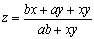.

Its extreme value is computed by setting both the partial derivative with respect to x and with respect to y equal to 0: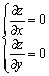, or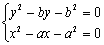.

Solving these equations simultaneously yields the positive solution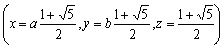,

corresponding to a saddle point. The z-value is the golden number, independently of the value of a and b. (This could have been expected, as an initial division by a and by b would have simply rescaled the problem.) The saddle point corresponds to maximum provided that x=y, and this leads to the special case of extending a square by another square to form a larger square: the added area is maximal, with respect to the area of the two squares, if the two non-square rectangles are golden rectangles (Figure 7).

The silver section can be obtained through such an optimisation procedure, but we summarise it in a somewhat simplified construction, starting with a square (a=b=1 ). Now, the area cut off at one side is compared to the total area of the two squares. The function to be maximised is therefore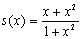.

The derivative corresponds to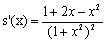,

and the silver mean is the positive solution of (Figure 8).

The given formulation corresponds to what is most probably intended by the expression of "optimal solution", as given by so many authors, and refuted by others. It proposes a rectangle that is neither too small nor too large, with respect to a given verifiable objective criterion. It seems but a rephrasing exercise, using mathematical vocabulary, but it could help to reboot the mathematical career of the golden section.

It is another matter to state that the given criterion produces the most elegant rectangle. Nevertheless, psychological golden section tests may be reformulated as "finding the rectangle such that the added area is maximal when compared to two squares constructed on the diagonal". People already have difficulties in choosing the right shape of a rectangle simply because estimating sizes is not easy. This obstacle may be important when making judgements about elegance of shapes and sizes.

REFERENCES

Brown, Sheldon. Sturmey-Archer Bicycle Hubs (web site), http://www.sheldonbrown.com/sturmey-archer.html

Campbell, S. L. and C. D. Meyer, Jr. 1979. Generalized Inverses of Linear Transformations. London: Pitman Publishing Limited.

Green, Christopher D. 1995. All that Glitters: A Review of Psychological Research on the Aesthetics of the Golden Section. Perception 24: 937-968.

Huylebrouck, D. 2001. Similarities in irrationality proofs for p, ln2, z(2) and z(3), Pp. 222-231 in The American Mathematical Monthly 108, 3: 222-231. (Cf. http://www.maa.org/pubs/monthly_mar_01_toc.html.)

Huylebrouck, D. and P. Labarque. 2000. Symmetric partition of colours on a rotating disk and the golden number. Pp. 217-218 in Abstracts Alhambra 2000. ALHAMBRA 2000: A Joint Mathematical European-Arabic Conference, July 3-7, Granada, Spain.

Huylebrouck, D. and P. Labarque. 2001. Colourful Errors. Paper presented at symposium, Mathematics & Design 2001. Third International Conference, July 3-7, Melbourne, Australia.

Huylebrouck, D. and P. Labarque. Golden grey. Submitted for publication.

Markowsky, George. 1992. Misconceptions about the Golden Ratio. The College Mathematics Journal 23, 1: 2-19.

Néroman, D. 1983. Le Nombre d' Or, à la portée de tous. Paris: Ariane.

Neveux, Marguerite. 1995. Le Mythe du Nombre d'Or. La Recherche 278, 26: 810-816.

Sharp, John. 2002. Spirals and the Golden Section, Nexus Network Journal 4, 1 (Winter 2002).

Spinadel, Vera W. de. 1998. From the Golden Mean to Chaos. Buenos Aires, Argentina: Nueva Libreria.

Sturmey-Archer internal hub gearing (web site), http://www.sturmey-archer.com/p20.htm.

Williams, Kim. 2001. The Shape of Divinity. The Mathematical Intelligencer 23, 1: 54-57.

RELATED SITES ON THE WWW

Dirk Huylebrouck

Patrick Labarque

 The correct citation for this article is:Dirk Huylebrouck and Patrick Labarque, "More True Applications of the Golden Number", Nexus Network Journal, vol. 4, no. 1 (Winter 2002), http://www.nexusjournal.com/Huy-Lab.html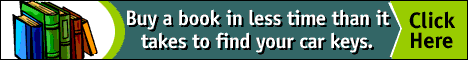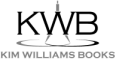top of page

NNJ Homepage

NNJ Winter 2002 Index

Related Sites on the WWW

Order books!

Research Articles

The Geometer's Angle

Didactics

Book Reviews

Conference and Exhibit Reports

The Virtual Library

Submission Guidelines

Top of Page